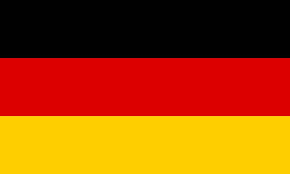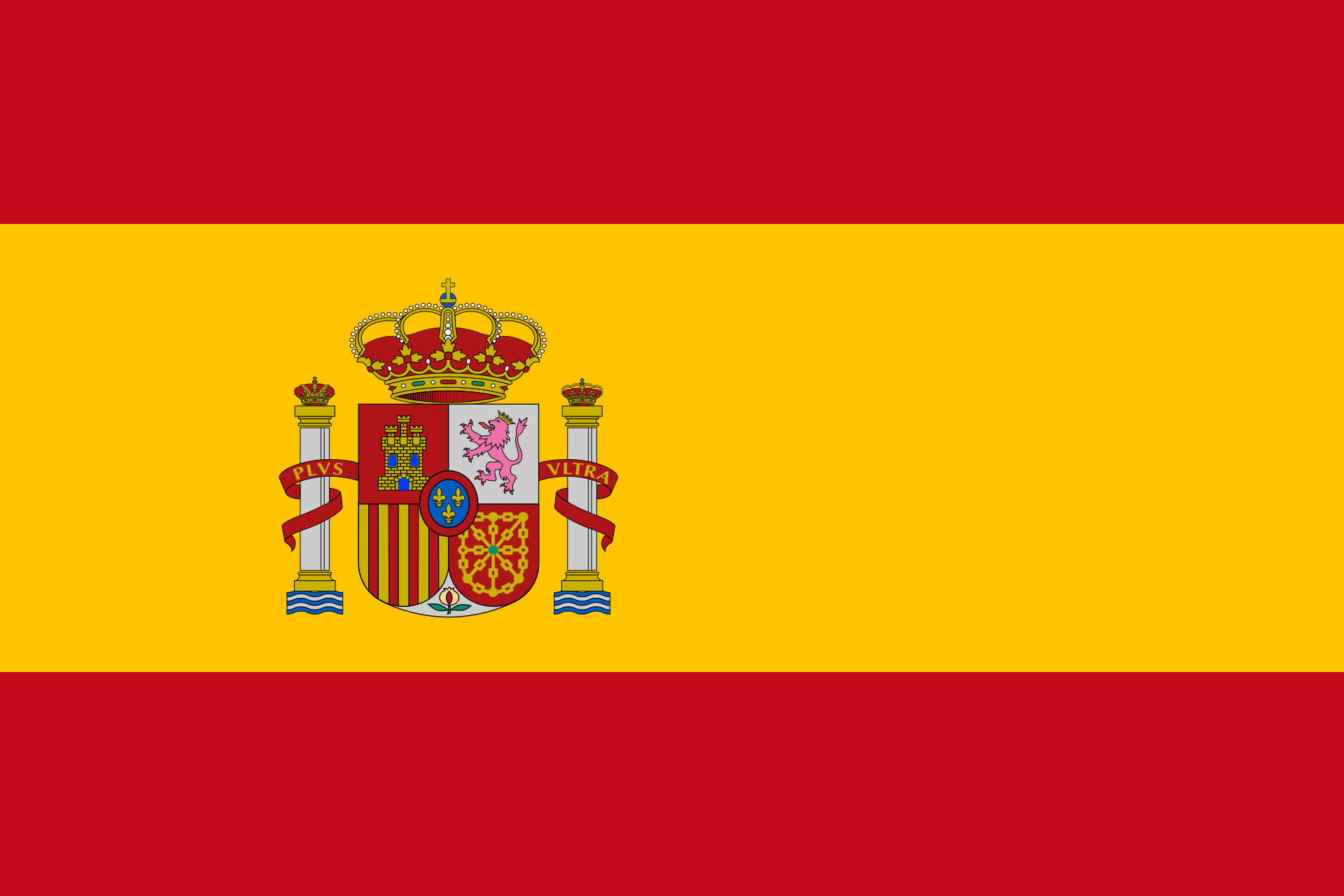# Plane intersectionChoose how the first plane is given.
• parametric equation:

 E: x =+ r+ s• Coordinate form:

• Point-normal form:

 E: (x-)⋅=0
• Given through three points

Intersection with plane

Choose how the second plane is given.
• parametric equation:

 E: x =+ r+ s• Coordinate form:

• Point-normal form:

 E: (x-)⋅=0
• Given through three points

## What's this about?

Imagine you got two planes in space. They may either intersect, then their intersection is a line. Or they do not intersect cause they are parallel. By equalizing plane equations, you can calculate what's the case. This gives a bigger system of linear equations to be solved.

## And how do I find out if my planes intersect?

Just enter them into our free calculatorģ